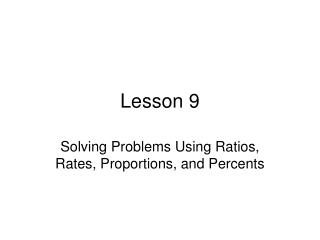Download PresentationLesson 9Lesson 9 - PowerPoint PPT Presentation

Download PresentationLesson 9
An Image/Link below is provided (as is) to download presentation

Download Policy: Content on the Website is provided to you AS IS for your information and personal use and may not be sold / licensed / shared on other websites without getting consent from its author. While downloading, if for some reason you are not able to download a presentation, the publisher may have deleted the file from their server.

- - - - - - - - - - - - - - - - - - - - - - - - - - - E N D - - - - - - - - - - - - - - - - - - - - - - - - - - -
Presentation Transcript

1. Lesson 9 Solving Problems Using Ratios, Rates, Proportions, and Percents

2. Ratio vs Proportions • A ratio is used to compare two quantities. They are sometimes used to compare parts to parts. For example, the ratio of boys to girls is 7 to 3. (7:3 or 7/3) • When two ratios are set equal to one another, they create a proportion. You can also use proportions to solve geometry problems, like when we solve similar figures or scale drawings. For example, 7/10 =14/20

3. Ratio and Proportion Practice • A bag contains only red and blue marbles. The ratio of blue marbles to red marbles in the bag is 2 to 3. If there are a total of 15 marbles in the bag, how many marbles in the bad are red. • If the ratio is 2 to 3, how many marbles are in the bag? • What is the next step to figure out how many total marbles in the bag?

4. Rate • A rate is a ratio that compares two different kinds of units. For example, 20 miles per hour is a rate that compares miles to hours.

5. Like this… • Hector paid \$31.41 for 4.5 pounds of chicken salad at a deli. At that rate, how much would it cost to buy 2 pounds of chicken salad? • We are going to use a ratio, proportion and a rate. What do you think we need to do to figure out how much he spent on 2 pounds? • How should we write our answer?

6. Percents • A percent is a ratio of a number to 100. For example, 25% can be written as the ratio 25/100. You can use proportions to solve percent problems, but we are going to practice using equations also.

7. Finding a percent • Elmo bought a CD that cost \$17.00. He also paid \$1.19 in sales tax. What is the percent of the sales tax? • Strategy 1- Set up a ratio of sales tax to total 1.19/17.0. Then we can set up a proportion to find the percent. Remember a percent is a number out of 100. • Strategy 2- We can set up an equation to solve: (part/whole) x 100.

8. Lets try another • A company that makes batteries check 5,000 batteries it recently produced. It found that 25 of the batteries were defective. What percent of the total batteries checked were defective. • Use both strategies. You will get the same answer.

9. More percents! • Sometimes, we need to use a percent to find a part of something. • A computer costs \$1,450. Sales tax is 6%. How much will he pay in sales tax? • Strategy 1- set up a proportion: %/100 = part/whole. • Strategy 2- write an equation. We have the percent and whole. Percent x Whole = Part • Enrich yourself: How much would the computer cost? • You can: Add the part to the whole to get the answer • Since percents are out of 100, 100% of the price is \$1,450. We are adding 6% sales tax. So 100+6 =106%. Take it from there.

10. One more type of problem • Shelly bought 6 cans of sauce. This is 40% of what is on the shelf. How many cans were on the shelf? • Strategy 1- Set up the proportion: %/100 = is/of or 40/100= 6/x • Strategy 2- Change the percent to a decimal. Divide the whole by the decimal. That is you answer.

11. Lets practice what we learned! • A team won 2 out of 5 games it played last season. If the team played a total of 35 games last season, how many games were won? • Kaz can run 5 kilometers in 30 minutes. At that rate, how far could Kaz run in 12 minutes? • Mr. Beyda ordered 200 new books for the school library. If 15 of the books he ordered are biographies, what percent of the books ordered are biographies?

12. Some more • The ratio of boys to girls in the school chorus is 6 to 8. If there are a total of 49 students in the chorus, how many boys are in the chorus? • The owner of a clothing store is selling a jacket for 175% of it wholesale price. If the wholesale price of a jacket is \$25, how much will the clothing store sell it for? • If Sarah pays only \$45 of her \$5,000 credit card bill, what percentage of the bill will she pay?

13. You know the drill… • A computer system that regularly sells for \$1,200 is on sale for 25% off. Tristan buys the computer system on sale and pays \$58.50 in sales tax. • What was the percent of sales tax? • Show each step of the work you did to solve this problem.